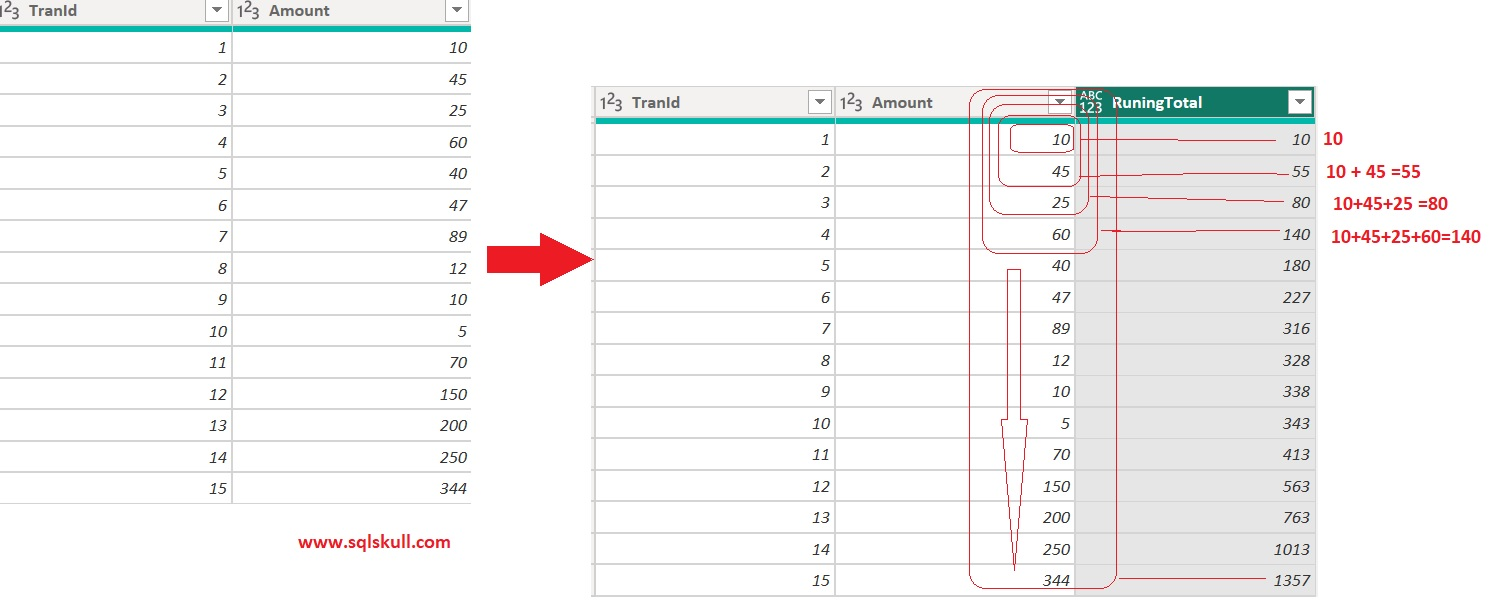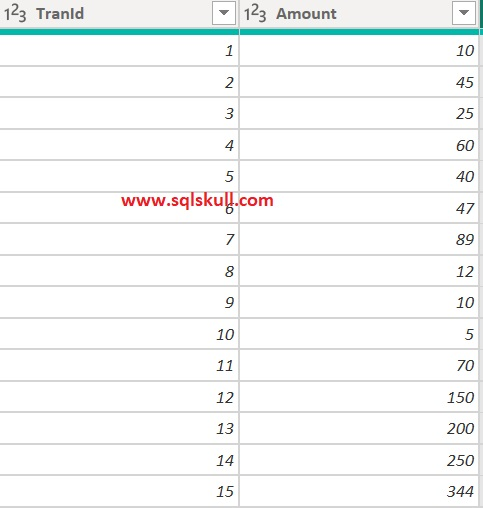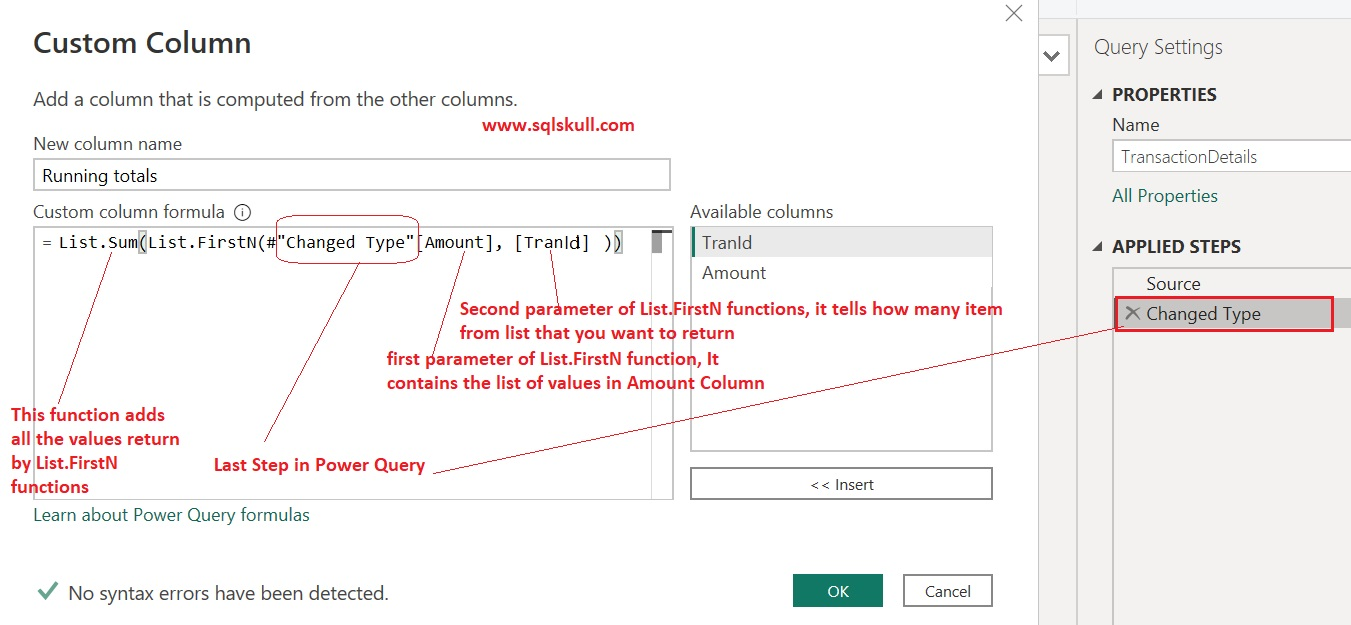Home » Running totals in Power Query

# Running totals in Power QueryA running totals or cumulative totals is a sequence of partial sum of values in any given table.

It just add up the values as you go, so for any current row the running total would be the total sum of all previous value including current row value as shown in below screenshot.

You can see, for first row the running total would be the sum of all previous, as there is no previous row so it would be zero 0 plus including current row value that is 10 so the cumulative total for first row would be 0+10=10.

For second row, the running total would be the total sum of all previous values that is 10, plus including current row value that is 45. So the running total for second row would be 10+45=55. In similar way, running total is calculated for remaining rows.Let’s see how to create a running totals in Power Query.

As you can see, here we have a sample data as shown below.Before proceeding further make sure you must have one unique column or index column in your table and all values in that column should be sequence such as 1, 2,3,4.. and so on.. In our case, we have TranId column in table.

We, will also see how to create a running totals if you do not have unique column in your table.

Let’s add custom column, In Power Query editor click on Add columns tab then click on Custom Column tab as shown below.Now, a pop up window appears now write the below M query code for custom column.

`List.Sum(List.FirstN(#"Changed Type"[Amount], [TrandId]))`

Note that, Changed Type is the last step created in Power Query so it could be different in your case.Once you create the custom column, you can see the running totals.Let’s understand the working of above code.

Here List.FirstN function used which returns the first set of items in the list by specifying how many items to return or a qualifying condition.

This function takes two parameter values, first is a list of values in our case it is Amount as we want running totals of values are Amount column.

Second parameter value is a condition or number which tells how many items that we want to return from list. So, here we have provided a TranId column which contains sequential values.

In case, if a value in TranId column is 5 that means List.FirstN function returns the first 5 amount values from list that is 10, 45, 25, 60, and 40.

Now, after that List.Sum function will adds all those values returned by List.FirstN function and return the final result that is 180, therefore the cumulative totals for 5th rows or first 5 rows will be 180.

Now we will see, how to create a running totals if you do not have unique column or index column in table.

Here we have taken a same table but without any unique column.Now create a index column in Power Query as shown below.

Click on Add Column tab then go to Index Column tab and select Options.

Here we have selected the Option From 1 as we want to create a index starting from 1.Now you can see, it creates an index column in table. All the values would be in sequence starting from 1.

Now you can create a running totals as we have done above.

Group By the data using Power Query

Create Index column using Power Query

Merge Queries

Import text using examples feature Power BI

Pivot columns – turn rows into columns

Split Columns By Positions## 1 thought on “Running totals in Power Query”

This site uses Akismet to reduce spam. Learn how your comment data is processed.